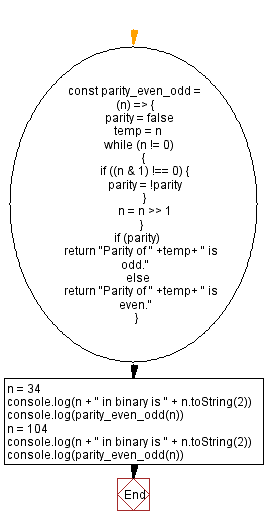# JavaScript - Parity of a given number

## JavaScript Bit Manipulation: Exercise-13 with Solution

Write a JavaScript program to calculate and find the parity of a given number.

In mathematics, parity is the property of an integer of whether it is even or odd. In binary numbers, parity refers to the total number of 1s. The odd parity(1) represents an odd number of 1s, whereas the even parity(0) represents an even number of 1s.
In information theory, a parity bit appended to a binary number provides the simplest form of error detecting code. If a single bit in the resulting value is changed, then it will no longer have the correct parity: changing a bit in the original number gives it a different parity than the recorded one, and changing the parity bit while not changing the number it was derived from again produces an incorrect result. In this way, all single-bit transmission errors may be reliably detected. Some more sophisticated error detecting codes are also based on the use of multiple parity bits for subsets of the bits of the original encoded value.

Test Data:
(34) -> "Parity of 34 is even."
"34 in binary is 100010" // Even number of 1s
(104) -> "Parity of 104 is odd."
"104 in binary is 1101000" // Odd number of 1s

Sample Solution:

JavaScript Code:

``````const parity_even_odd = (n) => {
parity = false
temp = n
while (n != 0)
{
if ((n & 1) !== 0) {
parity = !parity
}
n = n >> 1
}
if (parity)
return "Parity of " +temp+ " is odd."
else
return "Parity of " +temp+ " is even."
}
n = 34
console.log(n + " in binary is " + n.toString(2))
console.log(parity_even_odd(n))
n = 104
console.log(n + " in binary is " + n.toString(2))
console.log(parity_even_odd(n))
```
```

Sample Output:

```34 in binary is 100010
Parity of 34 is even.
104 in binary is 1101000
Parity of 104 is odd.
```

Flowchart:Live Demo:

See the Pen javascript-bit-manipulation-exercise-12 by w3resource (@w3resource) on CodePen.

* To run the code mouse over on Result panel and click on 'RERUN' button.*

Improve this sample solution and post your code through Disqus

What is the difficulty level of this exercise?

Test your Programming skills with w3resource's quiz.

﻿